# AA=7,BB=1 4) The mass of the system is AA kg and suspended by 3 cables. Find...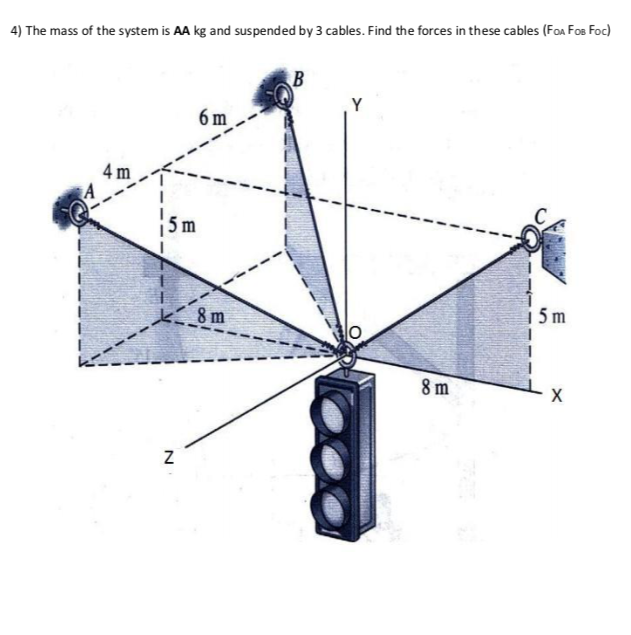AA=7,BB=1

4) The mass of the system is AA kg and suspended by 3 cables. Find the forces in these cables (Fon Fos Foc) B Y 6 m 4 m 5 m m k 8 m 15 m 8 8 m X N

The solution of the given problem is given below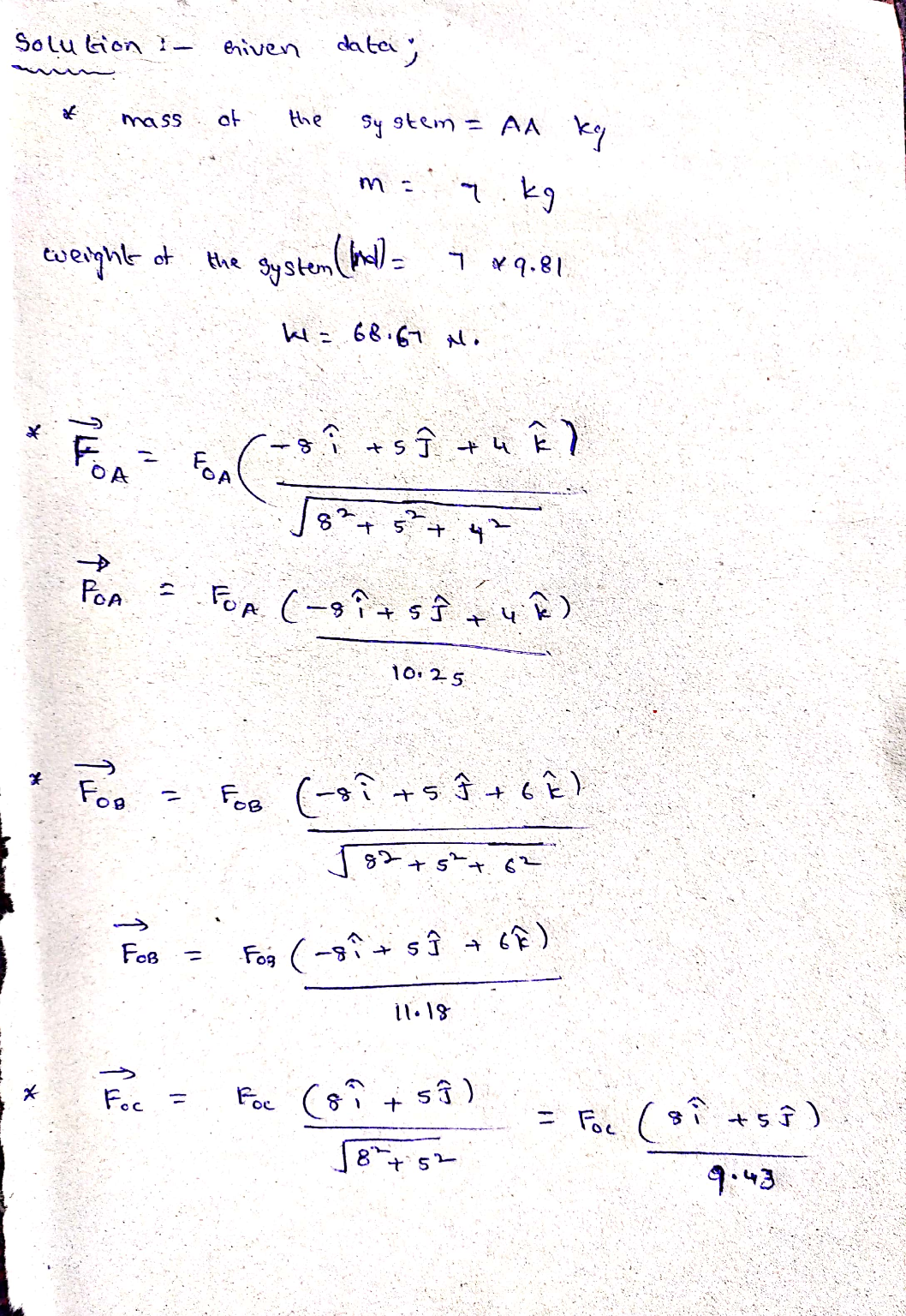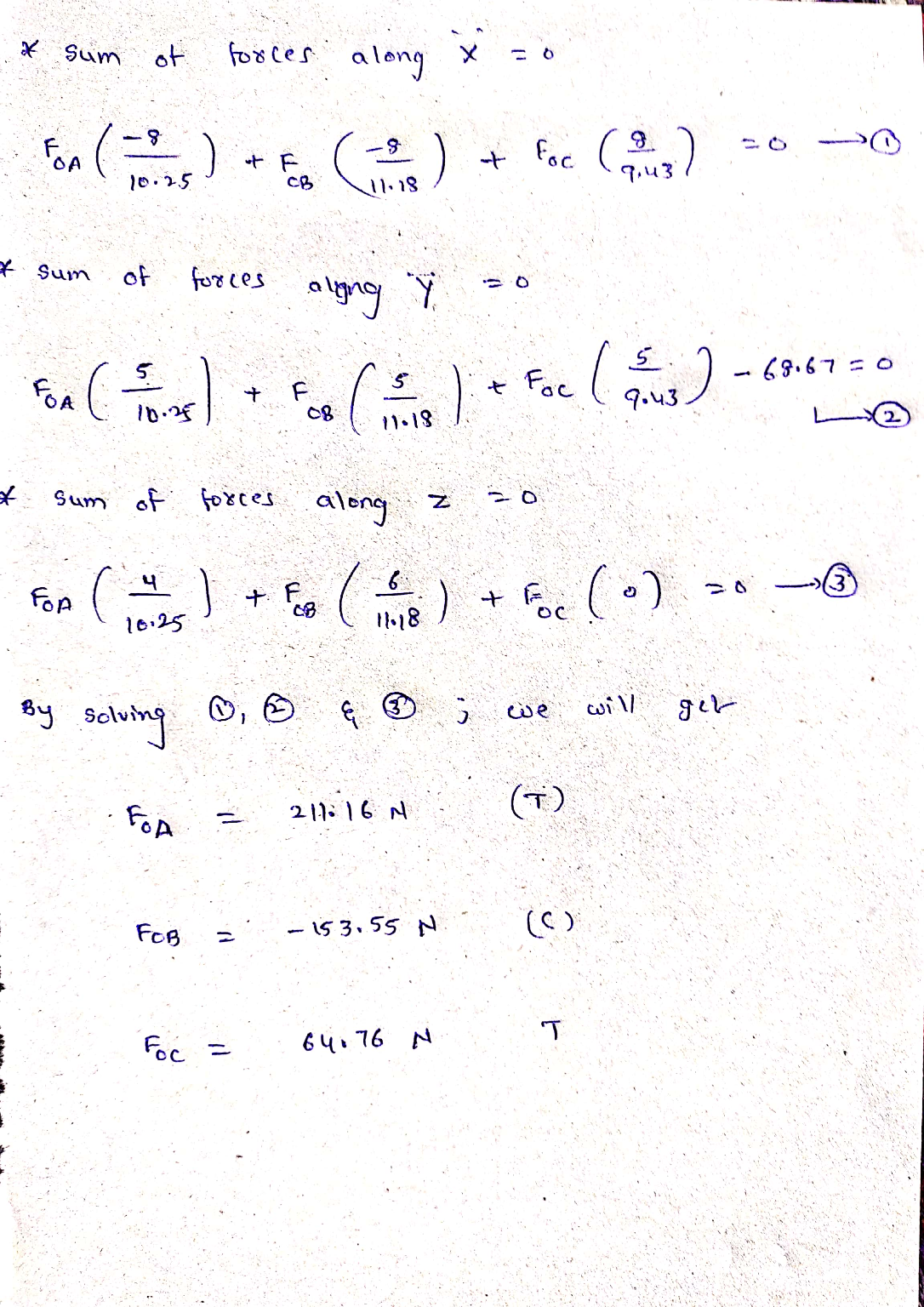##### Add Answer of: AA=7,BB=1 4) The mass of the system is AA kg and suspended by 3 cables. Find...
Similar Homework Help Questions
• ### AA=7,BB=1 1) If the system below is under equilibrium, find the forces passing from cable CA...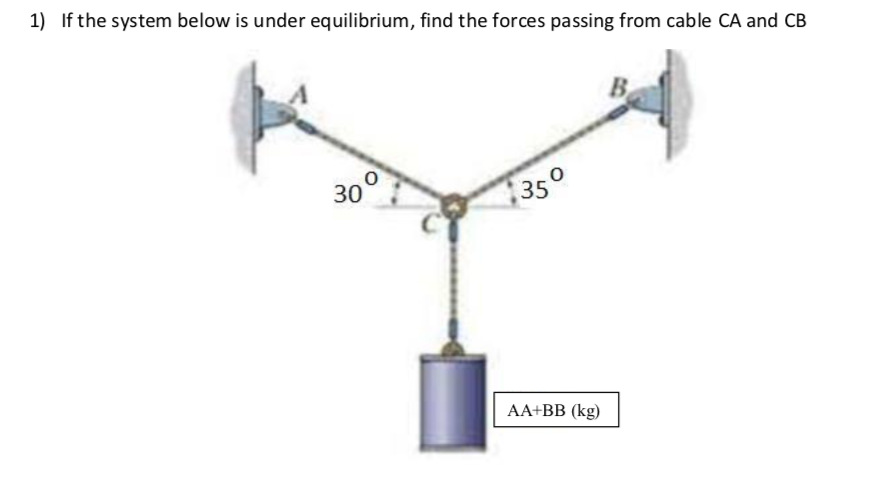AA=7,BB=1 1) If the system below is under equilibrium, find the forces passing from cable CA and CB 30 350 AA+BB (kg)

• ### AA=7,BB=1 3) For the system below a. Find the components of Fi, F and F; b....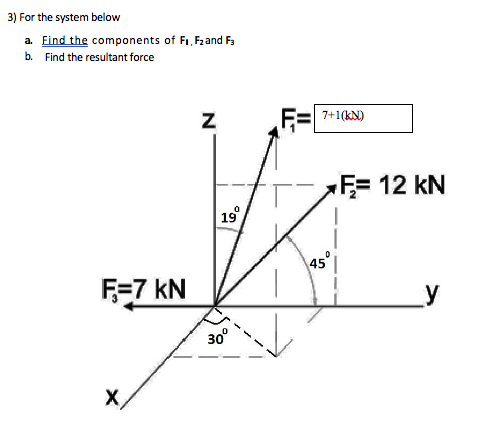AA=7,BB=1 3) For the system below a. Find the components of Fi, F and F; b. Find the resultant force N F=7+1(k) Ę= 12 kN 45° F=7 kN 30°

• ### A crate with a mass of 193.5 kg is suspended from the end of a uniform boom with a mass of 85.3 kg. The upper end of th...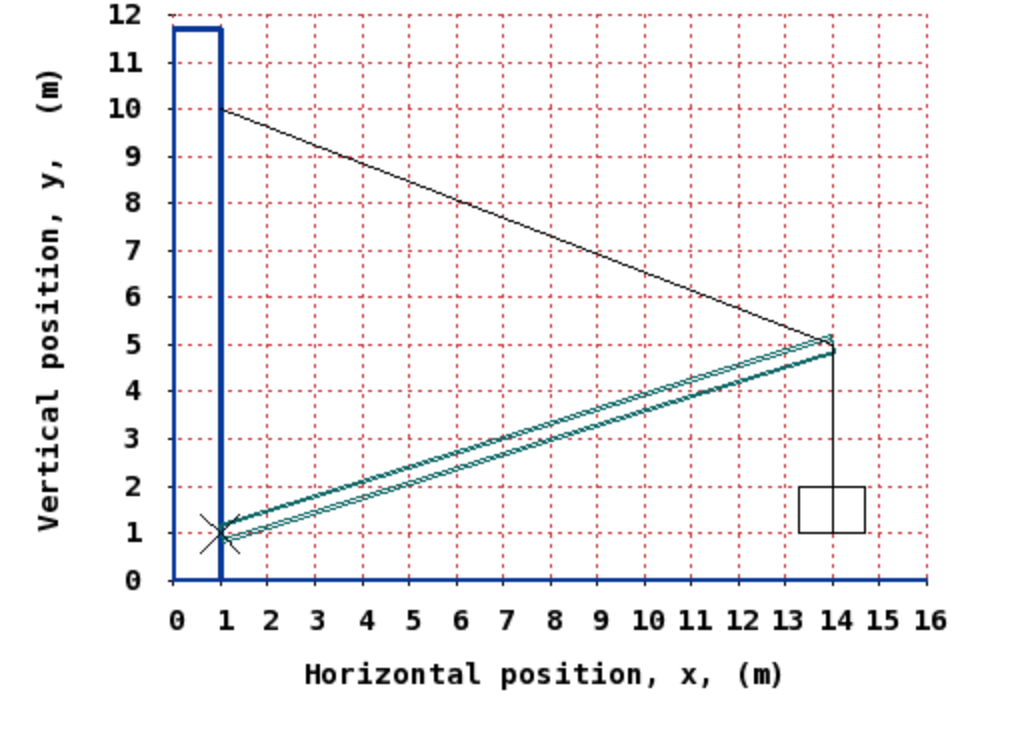A crate with a mass of 193.5 kg is suspended from the end of a uniform boom with a mass of 85.3 kg. The upper end of the boom is supported by a cable attached to the wall and the lower end by a pivot (marked X) on the same wall. Calculate the tension in the cable. 12 11 10 6 4 2 1 0 1 2 4 5 6 7 8 9 10 11 12 13 14 15 16...

• ### please include explanation A crate with a mass of 173.5 kg is suspended from the end...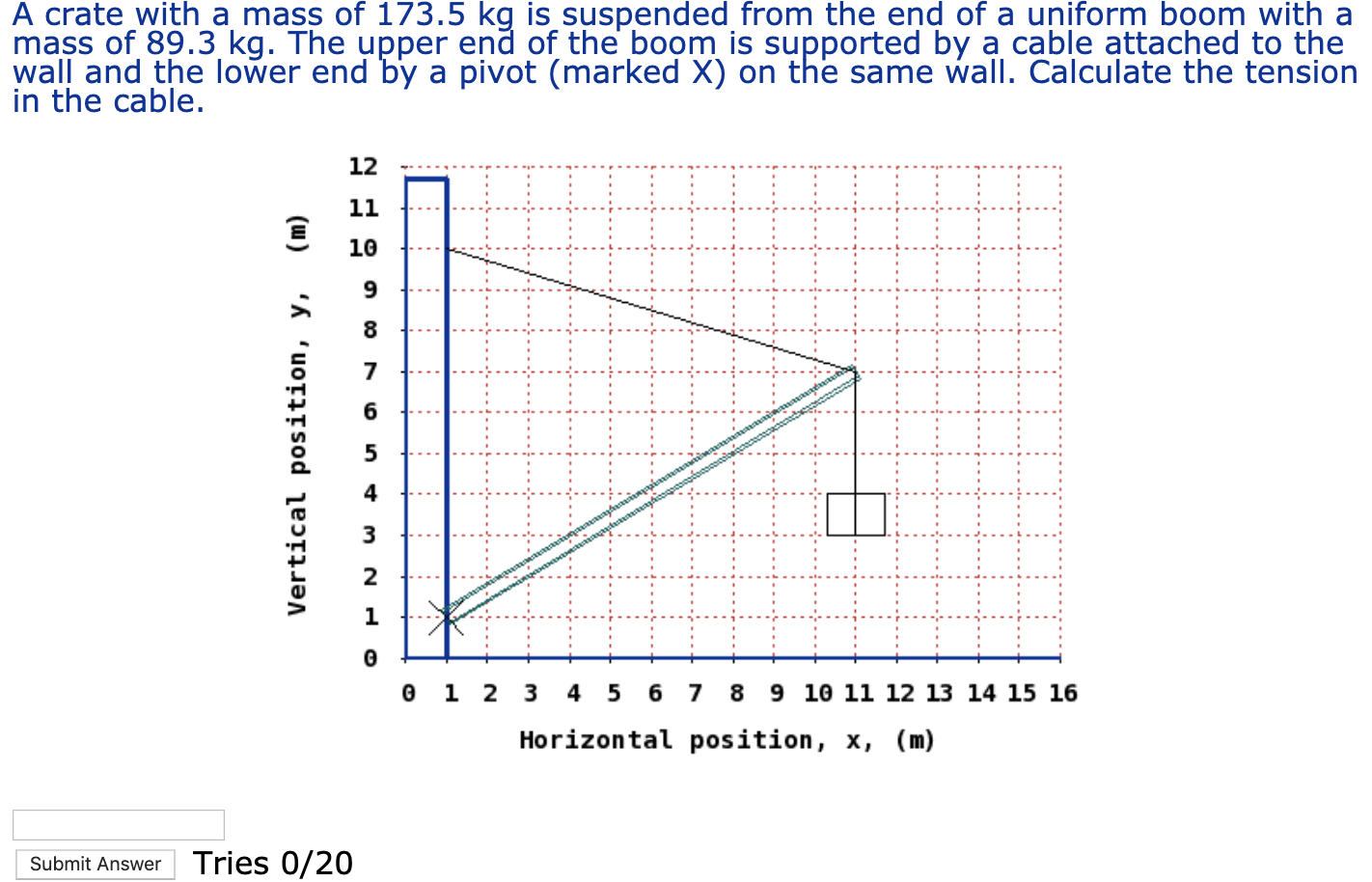please include explanation A crate with a mass of 173.5 kg is suspended from the end of a uniform boom with a mass of 89.3 kg. The upper end of the boom is supported by a cable attached to the wall and the lower end by a pivot (marked X) on the same wall. Calculate the tension in the cable. (m) Vertical position, y, OPNW Auovo EER HX 0 1 2 3 4 5 6 7 8 9 10 11...

• ### 4. A traffic light is suspended from two cables. In the figure below, the tension in...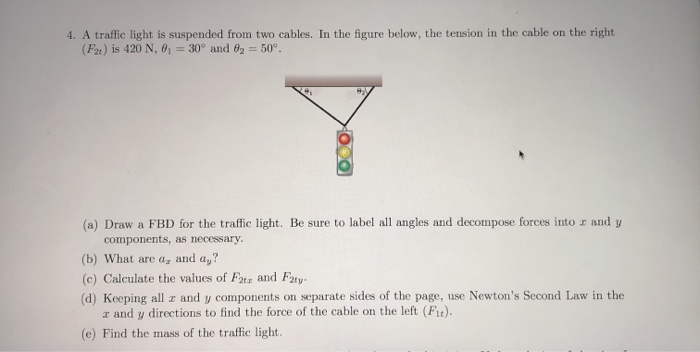4. A traffic light is suspended from two cables. In the figure below, the tension in the cable on the right (Ft) is 420 N, 0 = 30° and 82 = 50°. (a) Draw a FBD for the traffic light. Be sure to label all angles and decompose forces into r and y components, as necessary. (b) What are a, and ay? (c) Calculate the values of Far and Futy (d) Keeping all z and y components on separate sides...

• ### (d) A 4-kg mass is suspended from a spring with a constant k 25, and a dashpot with various level...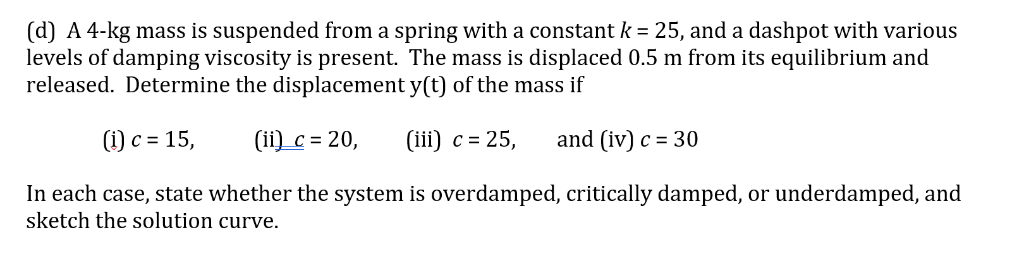(d) A 4-kg mass is suspended from a spring with a constant k 25, and a dashpot with various levels of damping viscosity is present. The mass is displaced 0.5 m from its equilibrium and released. Determine the displacement y(t) of the mass if (i) c-15 i) c20, (iii) c-25, and (iv) c 30 In each case, state whether the system is overdamped, critically damped, or underdamped, and sketch the solution curve. (d) A 4-kg mass is suspended from a...

• ### 1. (10 pts) A uniform 30.0-kg scaffold of length 3.0 m is supported by two light cables, as shown in the figure. A painter A of mass "m" kg stands 0.5 m from the left end of the scaffold....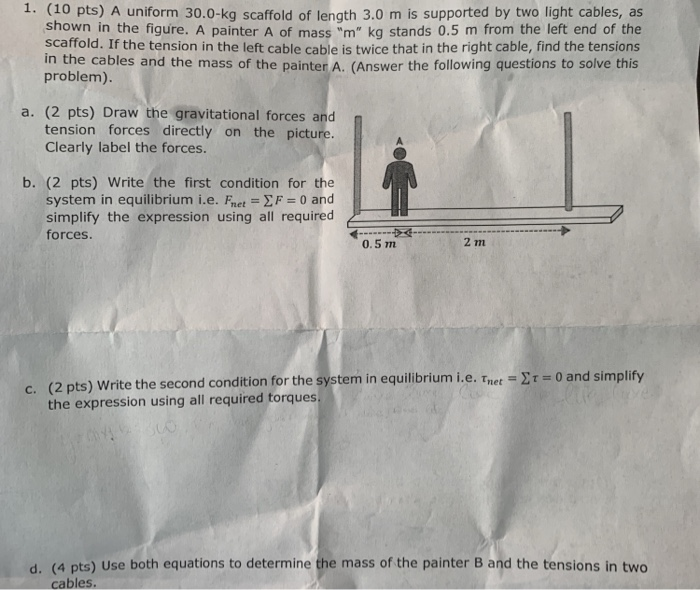1. (10 pts) A uniform 30.0-kg scaffold of length 3.0 m is supported by two light cables, as shown in the figure. A painter A of mass "m" kg stands 0.5 m from the left end of the scaffold. If the tension in the left cable cable is twice that in the right cable, find the tensions in the cables and the mass of the painter A. (Answer the following questions to solve this problem) a. (2 pts) Draw the...

• ### 010 10.0 points A weight (with a mass of 26 kg) is suspended from a point...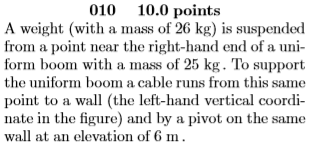010 10.0 points A weight (with a mass of 26 kg) is suspended from a point near the right-hand end of a uni- form boom with a mass of 25 kg. To support the uniform boom a cable runs from this same point to a wall (the left-hand vertical coordi- nate in the figure) and by a pivot on the same wall at an elevation of 6 m 10 9 8 25 kg 6 4 2 26 kg 012 3...

• ### 009 10.0 points A weight (with a mass of 100 kg) is suspended from a point...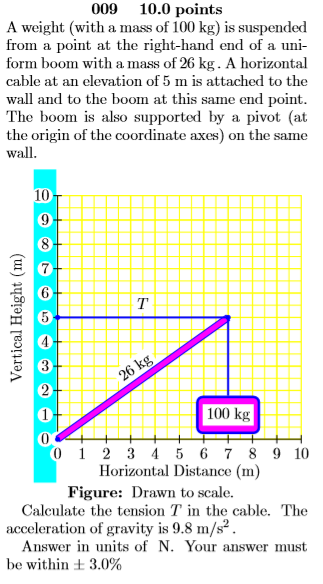009 10.0 points A weight (with a mass of 100 kg) is suspended from a point at the right-hand end of a uni form boom with a mass of 26 kg. A horizontal cable at an elevation of 5 m is attached to the wall and to the boom at this same end point The boom is also supported by a pivot (at the origin of the coordinate axes) on the same wall 10 7 6 т 26 kg 100...

• ### VALU.U POLUD A weight (with a mass of 79 kg) is suspended from a point near...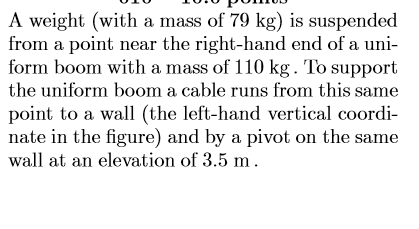VALU.U POLUD A weight (with a mass of 79 kg) is suspended from a point near the right-hand end of a uni- form boom with a mass of 110 kg. To support the uniform boom a cable runs from this same point to a wall (the left-hand vertical coordi- nate in the figure) and by a pivot on the same wall at an elevation of 3.5 m. 7 Vertical Height (m) 110 kg 79 kg 0 1 2 3 4...

Free Homework App